Time: 3 Hours                                                                                                     Max. Marks: 100

NOTE: There are 9 Questions in all.

·      Question 1 is compulsory and carries 20 marks. Answer to Q. 1. must be written in the space provided for it in the answer book supplied and nowhere else.

·      Out of the remaining EIGHT Questions answer any FIVE Questions. Each question carries 16 marks.

·      Any required data not explicitly given, may be suitably assumed and stated.

Q.1       Choose the correct or best alternative in the following:                                         (2x10)

a.       The correct order of the coordination number is SC, BCC, FCC and HCP unit cells is

(A)  12, 8, 12, 6.                                 (B)  6, 8, 12, 12.

(C)    8, 6, 12, 12.                                (D)  6, 12, 12, 8.

b.      Frankel and Schottky imperfections are

(A)    dislocations in ionic crystals.

(B)    grain boundaries in covalent crystals.

(C)    vacancies in ionic crystals.

(D)    vacancies in covalent crystals.

c.   The electronic polarizability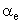of a mono atomic gas atom is

(A)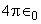(B)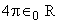(C)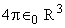(D)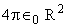d.   The forbidden energy gap of carbon in diamond structure is

(A)    0.7 ev                                         (B)  1.0 ev

(C)  0.01 ev                                        (D)  none

e.   For silicon doped with trivalent impurity,

(A)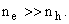(B)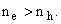(C)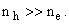(D)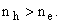f.    With increase in temperature, the orientation polarization in general

(A) decreases.                                     (B)  increases.

(C) remains same.                                (D) none of these.

g.   A suitable material for audio and TV transformers is

(A)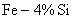.                                (B)  Ferrite.

(C)  Fe – 30% Ni.                               (D)  Pure Fe.

h.   Which of the following is not the function of oxide layer during IC fabrication

(A)    to increase the melting point of silicon.

(B)    to mask against diffusion or ion implant.

(C)    to insulate the surface electrically.

(D)    to produce a chemically stable surface.

i.    In normalizing, one of the following is not correct:

(A)   it relieves internal stresses              (B) it produces a uniform structure.

(C) the rate of cooling is rapid.             (D) the rate of cooling is slow.

j.    Which of the following materials is used for making permanent magnet.

(A)     Platinum cobalt                             (B) Alnico V

(C) Carbon Steel                                 (D) all the three

Answer any FIVE Questions out of EIGHT Questions.

Each question carries 16 marks.

Q.2     a.   What are the distinguishing characteristics of metallic bonding?  Discuss “cohesive energy”  and “electron affinity”.                                               (4+4)

b.   What do you understand by Miller indices of a crystal plane?  Show that in a cubic crystal the spacing between two consecutive parallel planes of Miller indices (hk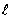) is given by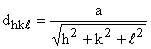.                                                           (3+5)

Q.3     a.   What are the point, line and surface imperfections found in solid materials?  Illustrate these imperfections with suitable sketches.                                 (9+3)

b.   What is the purpose of zone refining?  In a binary phase diagram (pressure omitted), what is the maximum number of phases that can coexist for at least one degree of freedom?                                (2+2)

Q.4     a.   State first and second Fick’s law of diffusion and show how it applies to diffusion which takes place in a solid solution.  What other factors influence the rate of such diffusion?                                         (8)

b.   What do you understand by “non-degenerate” and “degenerate” states?  Evaluate the temperature at which there is one percent probability that a state, with an energy 0.5 electron volt above the Fermi energy, will be occupied by an electron.                                                                                    (8)

Q.5     a.   Indicate on an energy level diagram the conduction and valence bands, donor & acceptor states and the position of fermi level for

(i)                  an intrinsic semiconductor.

(ii)                a n-type semiconductor.

(iii)               a p-type semiconductor.                                                             (6)

b.   What is Hall effect?  Briefly discuss the physical origin and uses of Hall effect?                    (2+5)

c.   Show that the probability of occupancy of energy level E by an electron is 50% for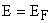at temperature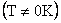.                                           (3)

Q.6     a.   Explain the following:

(i)  dielectric loss                                  (ii)  dielectric break down

(iii) local electric field                           (iv) polarizability.                          (2 ½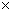4)

b.   The electrical resistivity of pure silicon is 2300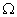at room temperature of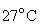, what will be its resistivity at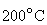.  (Take energy                        gap = 1.1 eV, K =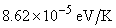.    (6)

Q.7     a.   Distinguish soft magnetic material from hard magnetic material in respect of hysteresis losses, eddy current losses & domain wall motion with suitable examples and plots.                                           (8)

b.   What are ferrites and ferrox cubes?  How are mixed ferrites prepared for industrial uses?  Give an account of the applications of ferrites pointing out their advantages over a ferromagnetic material.         (8)

Q.8     a.   What are the objectives of annealing?  Discuss the different annealing processes?  Is spheroidising different from annealing? Explain.                       (3+3+2)

b.   Distinguish with suitable examples & diagrams the following:

(i)                  Rolling and Forging.

(ii)                Extrusion and Wire drawing.                                                  (4+4)

Q.9           Describe briefly the following fabrication processes:

(i)                  Metallization.

(ii)                Photolithography.

(iii)               Single crystal growth.

(iv)              Casting.                                                                                          (44)